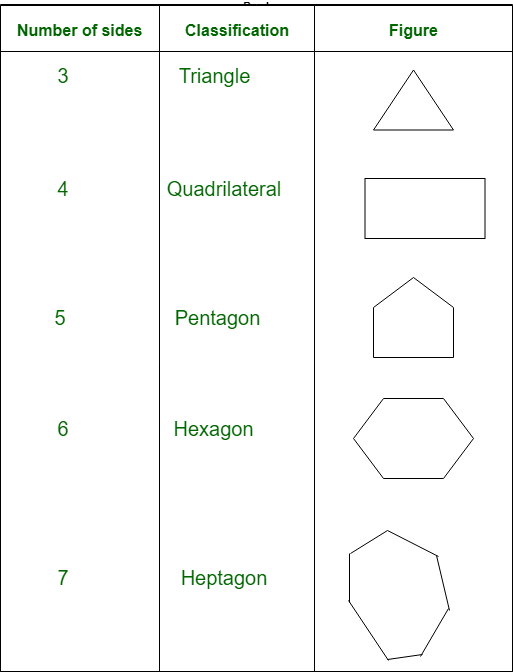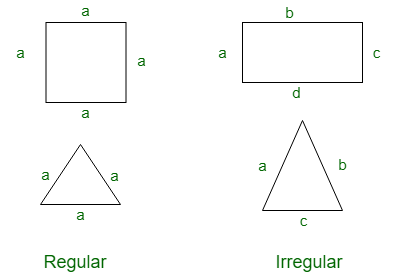# GRE Geometry | Polygons

Polygons:
The word polygon is derived from the Greek word polugonon, here poly means many and gons means angles. Polygons can be defined as a closed curve made up of line segments. Each line segment will become sides of the polygon.

Classification of polygons:
On the basis of the number of sides polygons are classified as follows:For n sides, we can call it n-gon. From here we can observe that the triangle is a polygon with least number of sides.

Concave and Convex polygon:
Convex polygons are the polygons whose all diagonal lie inside the figure and Concave polygons are those whose any diagonal lie outside the figure.Regular and Irregular polygon:
Regular polygons are the polygons having all sides of equal length and Irregular polygons are the polygons having different side length.Note:

• For a polygon, Exterior angle + Interior angle = 360°
• The sum of all exterior angles of polygon is 360°
• In a regular polygon all interior angles are equal.
• In a regular polygon all exterior angles are also equal.
• For a regular polygon, Number of sides = 360/exterior angle.
• Sum of all interior angles of a polygon is given by (n-2)*180, where ‘n’ is the number of sides in the polygon.
• Area of a polygon means area enclosed by the polygon and Perimeter of a polygon is sum of all the side length.

Examples:

• Example-1: What is the sum of all interior angles of heptagon ?
Solution:

```Since it is a Heptagon, number of sides (n) = 7
Sum of all interior angles = (n-1)*180 ```

So, for heptagon sum of interior angles = (7-2)*180 = 900

• Example-2: Calculate the number of side in a regular polygon having exterior angle 45° ?
Solution:

```Number of sides = 360/exterior angle
Since exterior angle is 45°
number of sides = 360/45 = 8 ```
• Example-3: What will be the value of an interior angle for a regular hexagon?
Solution:

```Since it is a hexagon, number of sides (n) = 6
exterior angle = 360°/6 = 60
we know that, interior angle + exterior angle = 180°
interior angle + 60° = 180°
interior angle = 180° - 60°
interior angle = 120 ° ```
• Example-4: Exterior angle of a regular polygon is 8°.
```(a) find the number of sides in the polygon?
(b) find the interior angle of the polygon? ```

Solution:

```(a) Number of sides = 360°/8°= 45
(b) interior angle = 180°- 8° = 172 ```
My Personal Notes arrow_drop_upCheck out this Author's contributed articles.

If you like GeeksforGeeks and would like to contribute, you can also write an article using contribute.geeksforgeeks.org or mail your article to contribute@geeksforgeeks.org. See your article appearing on the GeeksforGeeks main page and help other Geeks.

Please Improve this article if you find anything incorrect by clicking on the "Improve Article" button below.

Article Tags :

Be the First to upvote.

Please write to us at contribute@geeksforgeeks.org to report any issue with the above content.